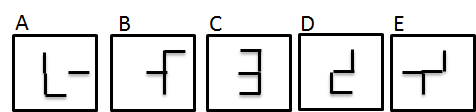### フィリピンスタッフの数値能力をあげるために行ってみたこと

フィリピン人の特徴の一つに数値に弱いというのがある。リーダークラスの数値が弱いと、数値目標の分解だったり、事業計画だったり、施策の結果分析だったり、数値を伴う仕事に大きな支障を生じる。そして実際に生じている。なので、リーダークラスの数値能力を向上させる必要がある。そのトレーニングとして、SPIのような算数をやらせるのがいいのじゃないかと思い、日本のSPIに使われるような問題を英訳してやってみた。

### Q.1

Five shapes are aligned according to a pattern. Which one (between A to E) can be in the blank?### Q.2

When a coin is tossed six times in succession, how many combinations are there that the coin lands head four times out of six?

### Q.3

I bought apples, oranges, and grapes, nine of them in total. Following is true.
i) At least I bought one for each fruit.
ii) I bought more apples than oranges.

(1) Which sentences (a-c) are always true?
a. If there are 3 apples, then there are 2 oranges.
b. If there are 3 oranges, then there are 2 grapes.
c. If there are 6 grapes, then there are 2 apples.

(2) Which sentences (d-f) are always true?
d. If there are 3 or less apples, then there are 4 or more grapes.
e. If the number of oranges is more than number of grapes, then there is one grape.
f. If the number of oranges and grapes are the same, then there are 7 apples.

### Q.4

In a box, there are 3 red balls, 2 yellow balls, and 1 blue ball.
Take 1 ball from the box, look at the color of a ball, and put it back in the box. We repeated it three times.

(1) What is the probability of taking a red ball in the first stage, yellow in the second stage, and blue in the third stage?

(2) What is the probability that a blue ball is picked exactly two times, and the second time occurs on the third draw?

### Q.5

We asked the visitors of the national parks P, Q, R, and S about their principal transportation.
Table 1 shows the percentage of each transportation for each park.
Table 2 shows the percentage of the number of each park’s respondents out of the total number of respondents.

[Table 1]

 P Q R S 4parks Car 60% 10% 10% 24% Bus 20% 40% 20% Train 10% 30% 30% 60% Other 10% 10% 20% 10% 14% TOTAL 100% 100% 100% 100% 100%

[Table 2]

 P Q R S 4parks Percentage of respondents 20% 10% 40% 30% 100%

(1) What is the occupied ratio of those who used a car at park P of the total respondents of 4 parks?

(2) What is the occupied ratio of those who used a train of the total respondents?

(3) What is the occupied ratio of those who used a car in park Q in relation to all the respondents of park Q?

### Q.6

The monthly fixed rate of a network circuit is ¥3,000. If usage exceeds 100 hours, ¥20 is imposed for every hour in excess of 100 hours. (¥20 / hour is imposed when the operating time exceeds 100 hours.)
When the average rate per hour in a month is ¥22, how many hours is the operating time of the month?

### Q.7

He got a new TV by paying in three installments. He paid one sixth of the total price on the first installment

(1) If he pays three-eighths of the total price on the second installment and pays the rest in the third installment, what percentage of the total price will he pay in the third installment? *There is no interest.

(2) If he pays two-thirds of the first installment on the second installment and pays the rest in the third installment, what percentage is the third installment of the first installment? *There is no interest.

### Q.8

We set the price of a product to gain 50% profit of the cost. However, it did not sell well, and we sold it at a 30% discount off the listed price. As a result, we gained ¥120 profit. How much is the cost of this product?

### Q.9

This is a time schedule of a train that leaves P station and goes to T station via Q station, R station, and S station.

P station departure 10:00

Q station arrival 10:20
departure 10:25

R station arrival 10:55
departure 11:00

S station pass

T station arrival 12:30

(1) If the average speed between P and Q is 108 km/hour, and the distance between P and R is 98 km, what is the average speed between Q and R?

(2) If the average speed between R and S is 110 km /hour, between S and T is 130 km /hour, and the distance between R and T is 187km, at what time does the train pass S station?

1. コメント 0

1. トラックバック 0